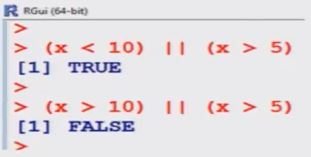# Basic calculations: Missing data and logical operators in R Language

Missing data

R represents missing observations through the data value NA
We can detect missing values using is.na

> x  <-  NA             # assign NA to variable x
> is.na (x)               # is it missing ?
    TRUE

Now try a vector to know if any value is missing?

> x <-  c(11, NA, 13)
> is.na (x)
 FALSE TRUE FALSE

Example : How to work with missing data

> x  <-  c(11, NA, 13)  # vector
> mean (x)     11 + NA + 13/2
   NA
> mean (x, na.rm = TRUE )  # NAs can be removed
  12
11 + 13/2 = 12
The null object, called NULL, is returned by some functions and expressions.

Note that NA and NULL are not the same.

NA is a placeholder for something that exists but is missing.

NULL stands for something that never existed at all.

Logical Operators and Comparisons

The following table shows the operations and functions for logical comparisons (True or False)

TRUE and FALSE are reserved words denoting logical constants.

Logical Operators and Comparisons

• The shorter form performs element-wise comparisons in almost the same way as arithmetic operators.
• The longer form evaluates left to right examining only the first element of each vector. Evaluation proceeds only until the result is determined.
• The longer form is appropriate for programming control-flow and typically preferred in if clauses (conditional).
TRUE and FALSE are reserved words denoting logical constants

Example

> x  <- 5
Is x less than 10 or x is greater than 5 ?
> (x < 10) | | (x > 5)   # | | means OR
  TRUE

Is x greater than 10 or x is greater than 5 ?
> (x > 10) | |  (x > 5)
 FALSE